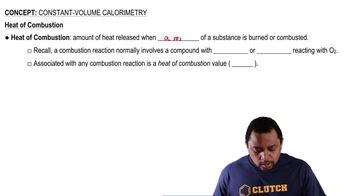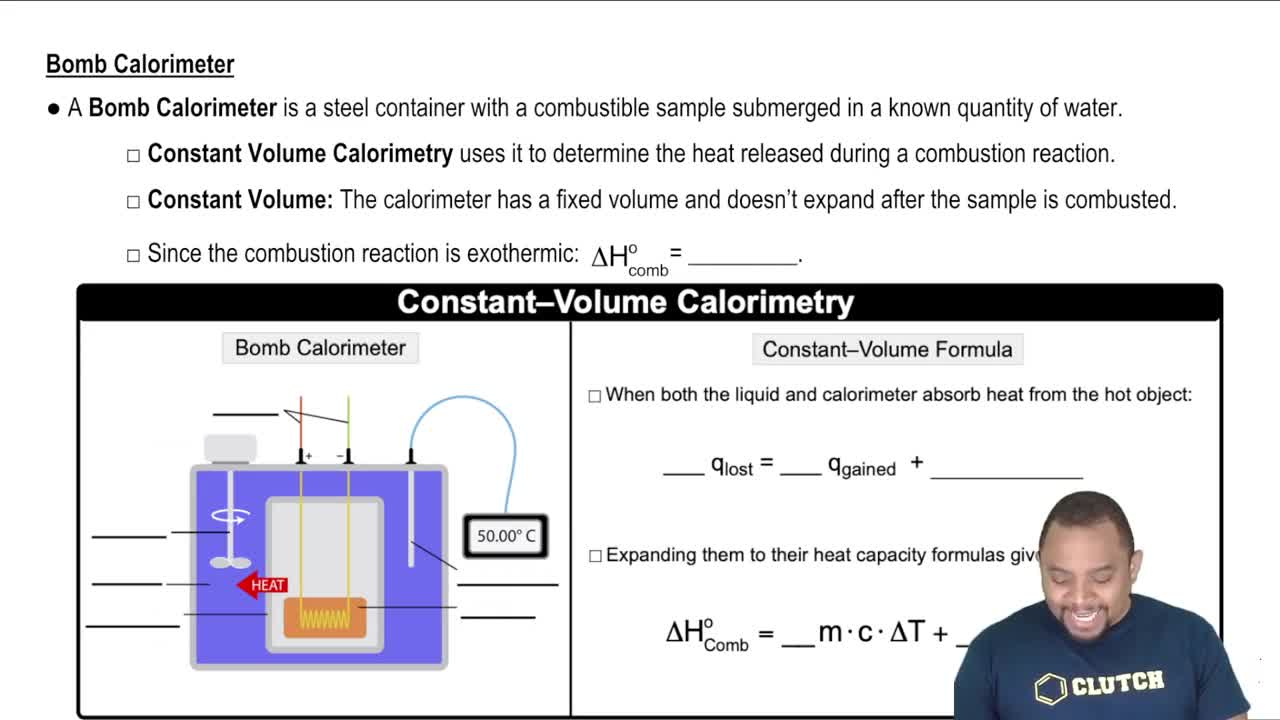Start typing, then use the up and down arrows to select an option from the list.
4:08 minutes
Problem 107
Textbook Question

# (b) Assuming that there is an uncertainty of 0.002 °C in each temperature reading and that the masses of samples are measured to 0.001 g, what is the estimated uncertainty in the value calculated for the heat of combustion per mole of caffeine?Verified Solution
This video solution was recommended by our tutors as helpful for the problem above.
80views